# MetaTrader 5 Platform build 3390: Float in OpenCL and in mathematical functions, Activation and Loss methods for machine learning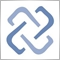Moderator
251846

The MetaTrader 5 platform update will be released on Thursday, August 4, 2022. The update will feature the following changes:

1. Terminal: Added automatic opening of a tutorial during the first connection to a trading account. This will assist beginners in learning trading basics and in exploring platform features. The tutorial is divided into several sections, each of which provides brief information on a specific topic. The topic completion progress is shown with a blue line.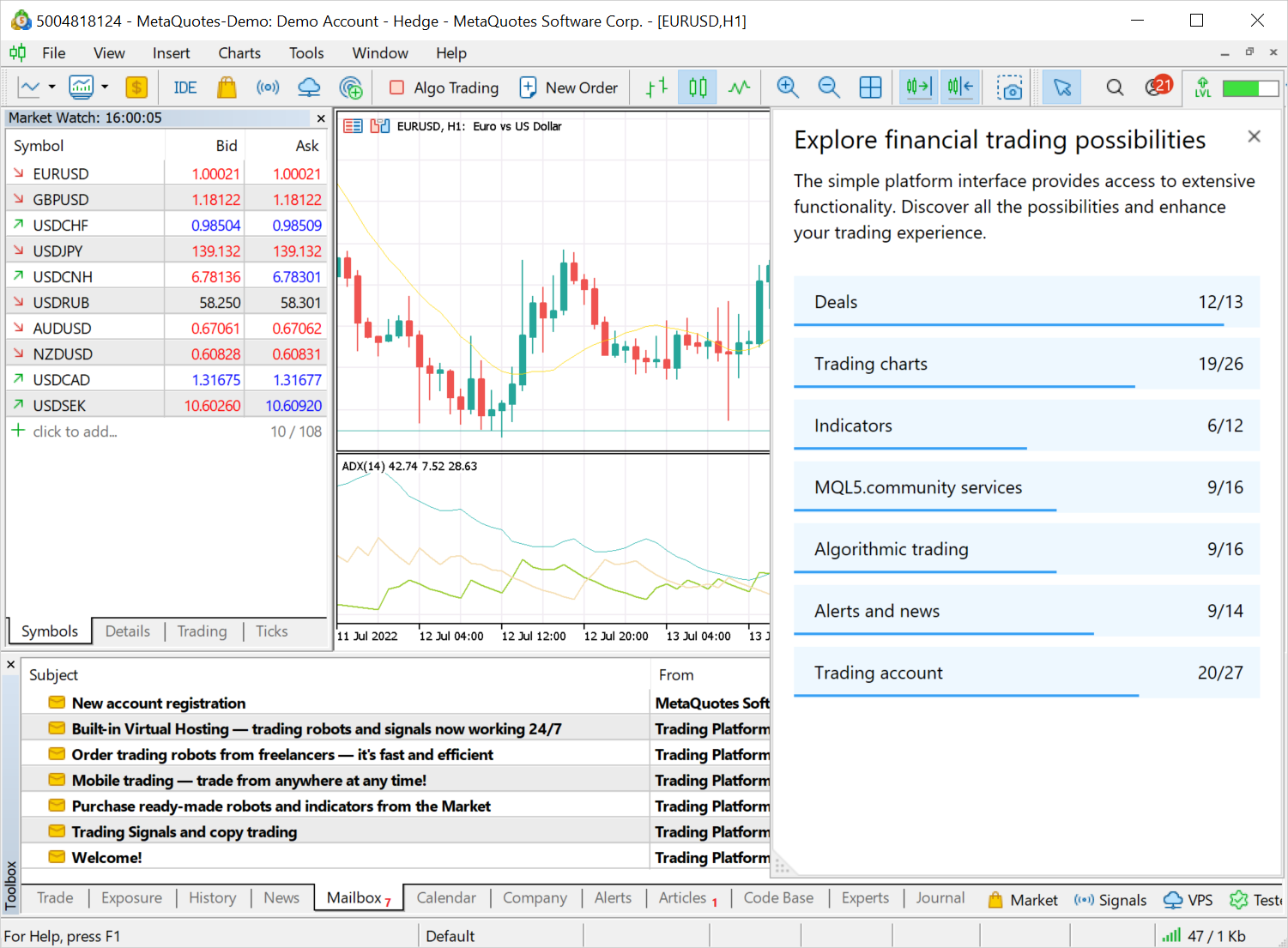2. Terminal: Fixed 'Close profitable'/'Close losing' bulk operations. Previously, the platform used opposite positions if they existed. For example, if you had two losing Buy positions for EURUSD and one profitable Sell position for EURUSD, all three positions would be closed during the 'Close losing' bulk operation. Buy and Sell would be closed by a 'Close by' operation, while the remaining Buy would be closed by a normal operation. Now the commands operate properly: they only close the selected positions, either profitable or losing.

3. Terminal: Fixed display of negative historical prices. Such prices will appear correctly for all timeframes.
4. Terminal: Optimized and significantly reduced system resource consumption by the terminal.
5. Terminal: Updated fundamental database for trading instruments. The number of data aggregators available for exchange instruments has been expanded to 15. Users will be able to access information on even more tickers via the most popular economic aggregators.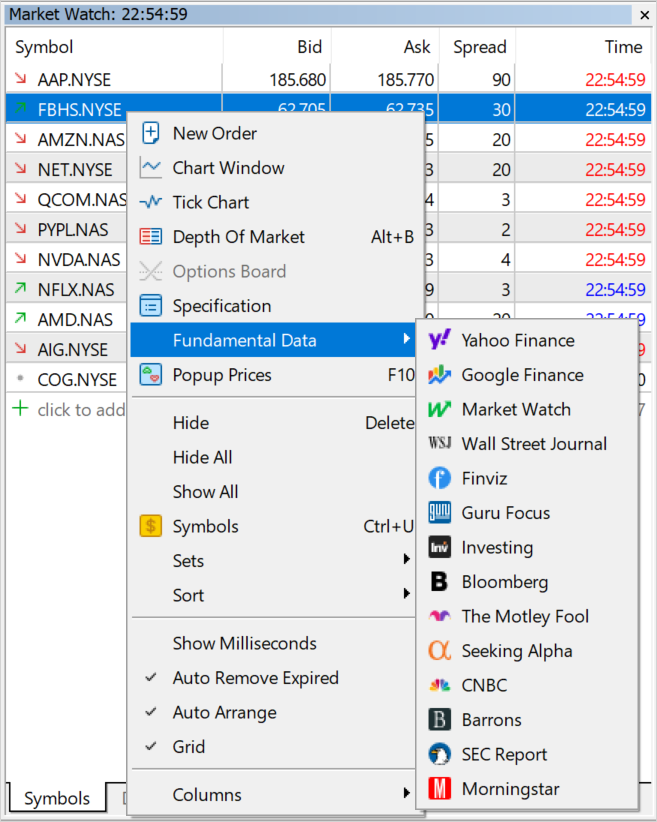About 7,000 securities and more than 2,000 ETFs are listed on the global exchange market. Furthermore, exchanges provide futures and other derivatives. The MetaTrader 5 platform offers access to a huge database of exchange instruments. To access the relevant fundamental data, users can switch to the selected aggregator's website in one click directly from the Market Watch. For convenience, the platform includes a selection of information sources for each financial instrument.

6. Terminal: Fixed Stop Loss and Take Profit indication in the new order placing window. For FIFO accounts, stop levels will be automatically set in accordance with the stop levels of existing open positions for the same instrument. This procedure is required to comply with the FIFO rule.

7. MQL5: Mathematical functions can now be applied to matrices and vectors.

We continue expanding algorithmic trading and machine learning capabilities in the MetaTrader 5 platform. Previously, we have added new data types: matrices and vectors, which eliminate the need to use arrays for data processing. More than 70 methods have been added to MQL5 for operations with these data types. The new methods enable linear algebra and statistics calculations in a single operation. Multiplication, transformation and systems of equations can be implemented easily, without extra coding. The latest update includes mathematical functions.

Mathematical functions were originally designed to perform relevant operations on scalar values. From this build and onward, most of the functions can be applied to matrices and vectors. These include MathAbs, MathArccos, MathArcsin, MathArctan, MathCeil, MathCos, MathExp, MathFloor, MathLog, MathLog10, MathMod, MathPow, MathRound, MathSin, MathSqrt, MathTan, MathExpm1, MathLog1p, MathArccosh, MathArcsinh, MathArctanh, MathCosh, MathSinh, and MathTanh. Such operations imply element-wise handling of matrices and vectors. Example:
```//---
matrix a= {{1, 4}, {9, 16}};
Print("matrix a=\n",a);

a=MathSqrt(a);
Print("MatrSqrt(a)=\n",a);
/*
matrix a=
[[1,4]
[9,16]]
MatrSqrt(a)=
[[1,2]
[3,4]]
*/```
For MathMod and MathPow, the second element can be either a scalar or a matrix/vector of the appropriate size.

The following example shows how to calculate the standard deviation by applying math functions to a vector.
```//+------------------------------------------------------------------+
//| Script program start function                                    |
//+------------------------------------------------------------------+
void OnStart()
{
//--- Use the initializing function to populate the vector
vector r(10, ArrayRandom); // Array of random numbers from 0 to 1
//--- Calculate the average value
double avr=r.Mean();       // Array mean value
vector d=r-avr;            // Calculate an array of deviations from the mean
Print("avr(r)=", avr);
Print("r=", r);
Print("d=", d);
vector s2=MathPow(d, 2);   // Array of squared deviations
double sum=s2.Sum();       // Sum of squared deviations
//--- Calculate standard deviation in two ways
double std=MathSqrt(sum/r.Size());
Print(" std(r)=", std);
Print("r.Std()=", r.Std());
}
/*
avr(r)=0.5300302133243813
r=[0.8346201971495713,0.8031556138798182,0.6696676534318063,0.05386516922513505,0.5491195410016175,0.8224433118686484,...
d=[0.30458998382519,0.2731254005554369,0.1396374401074251,-0.4761650440992462,0.01908932767723626,0.2924130985442671, ...
std(r)=0.2838269732183663
r.Std()=0.2838269732183663
*/
//+------------------------------------------------------------------+
//| Fills the vector with random values                              |
//+------------------------------------------------------------------+
void ArrayRandom(vector& v)
{
for(ulong i=0; i<v.Size(); i++)
v[i]=double(MathRand())/32767.;
}
```

8. MQL5: Added support in template functions for notations matrix<double>, matrix<float>, vector<double>, vector<float> instead of the corresponding matrix, matrixf, vector and vectorf types.

9. MQL5: Improved mathematical functions for operations with the float type. The newly implemented possibility to apply mathematical functions to 'float' matrix and vectors has enabled an improvement in mathematical functions applied to 'float' scalars. Previously, these function parameters were unconditionally cast to the 'double' type, then the corresponding implementation of the mathematical function was called, and the result was cast back to the 'float' type. Now the operations are implemented without extra type casting.

The following example shows the difference in the mathematical sine calculations:

```//+------------------------------------------------------------------+
//| Script program start function                                    |
//+------------------------------------------------------------------+
void OnStart()
{
//---  Array of random numbers from 0 to 1
vector d(10, ArrayRandom);
for(ulong i=0; i<d.Size(); i++)
{
double delta=MathSin(d[i])-MathSin((float)d[i]);
Print(i,". delta=",delta);
}
}
/*
0. delta=5.198186103783087e-09
1. delta=8.927621308885136e-09
2. delta=2.131878673594656e-09
3. delta=1.0228555918923021e-09
4. delta=2.0585739779477308e-09
5. delta=-4.199390279957527e-09
6. delta=-1.3221741035351897e-08
7. delta=-1.742922250969059e-09
8. delta=-8.770715820283215e-10
9. delta=-1.2543186267421902e-08
*/
//+------------------------------------------------------------------+
//| Fills the vector with random values                              |
//+------------------------------------------------------------------+
void ArrayRandom(vector& v)
{
for(ulong i=0; i<v.Size(); i++)
v[i]=double(MathRand())/32767.;
}```

10. MQL5: Added Activation and Derivative methods for matrices and vectors:
 AF_ELU Exponential Linear Unit AF_EXP Exponential AF_GELU Gaussian Error Linear Unit AF_HARD_SIGMOID Hard Sigmoid AF_LINEAR Linear AF_LRELU Leaky REctified Linear Unit AF_RELU REctified Linear Unit AF_SELU Scaled Exponential Linear Unit AF_SIGMOID Sigmoid AF_SOFTMAX Softmax AF_SOFTPLUS Softplus AF_SOFTSIGN Softsign AF_SWISH Swish AF_TANH Hyperbolic Tangent AF_TRELU Thresholded REctified Linear Unit
The neural network activation function determines how the weighted input signal sum is converted into a node output signal at the network level. The selection of the activation function has a big impact on the neural network performance. Different parts of the model can use different activation functions. In addition to all known functions, MQL5 also offers derivatives. Derivative functions enable fast calculation of adjustments based on the error received in learning.
11. MQL5: Added Loss function for matrices and vectors. It has the following parameters:

 LOSS_MSE Mean Squared Error LOSS_MAE Mean Absolute Error LOSS_CCE Categorical Crossentropy LOSS_BCE Binary Crossentropy LOSS_MAPE Mean Absolute Percentage Error LOSS_MSLE Mean Squared Logarithmic Error LOSS_KLD Kullback-Leibler Divergence LOSS_COSINE Cosine similarity/proximity LOSS_POISSON Poisson LOSS_HINGE Hinge LOSS_SQ_HINGE Squared Hinge LOSS_CAT_HINGE Categorical Hinge LOSS_LOG_COSH Logarithm of the Hyperbolic Cosine LOSS_HUBER Huber

The loss function evaluates the quality of model predictions. The model construction targets the minimization of the function value at each stage. The approach depends on the specific dataset. Also, the loss function can depend on weight and offset. The loss function is one-dimensional and is not a vector since it provides a general evaluation of the neural network.

12. Added matrix::CompareByDigits and vector::CompareByDigits methods for matrices and vectors. They compare the elements of two matrices/vectors up to significant digits.

13. Added support for MathMin and MathMax functions for strings. The functions will use lexicographic comparison: letters are compared alphabetically, case sensitive.

14. MQL5: The maximum number of OpenCL objects has been increased from 256 to 65536. OpenCL object handles are created in an MQL5 program using the CLContextCreate, CLBufferCreate and CLProgramCreate functions. The previous limit of 256 handles was not enough for the efficient use of machine learning methods.

15. MQL5: Added ability to use OpenCL on graphical card without 'double' support. Previously, only GPUs supporting double were allowed in MQL5 programs, although many tasks allow calculations using float. The float type is initially considered native for parallel computing, as it takes up less space. Therefore, the old requirement has been lifted.

To set the mandatory use of GPUs with double support for specific tasks, use the CL_USE_GPU_DOUBLE_ONLY in the CLContextCreate call.
```   int cl_ctx;
//--- Initializing the OpenCL context
if((cl_ctx=CLContextCreate(CL_USE_GPU_DOUBLE_ONLY))==INVALID_HANDLE)
{
return;
}```

16. MQL5: Fixed operation of the CustomBookAdd function. Previously, a non-zero value in the MqlBookInfo::volume_real field prevented the function from creating a Market Depth snapshot. The check is now performed as follows:
The transmitted data is validated: type, price and volume data must be specified for each element. Also, MqlBookInfo.volume and MqlBookInfo.volume_real must not be zero or negative. If both volumes are negative, this will be considered an error. You can specify any of the volume types or both of them, while the system will use the one that is indicated or is positive:

volume=-1 && volume_real=2 — volume_real=2 will be used,

volume=3 && volume_real=0 — volume=3 will be used.

Increased-precision volume MqlBookInfo.volume_real has a higher priority than MqlBookInfo.volume. Therefore, if both values are specified and are valid, volume_real will be used.

If any of the Market Depth elements is described incorrectly, the system will discard the transferred state completely.

17. MQL5: Fixed operation of the CalendarValueLast function. Due to an error, successive function calls after changes in the Economic Calendar (the 'change' parameter was set a new value after the call) could skip some events when using the currency filter.
`CalendarValueLast(change, result, "", "EUR")`
18. MQL5: Fixed ArrayBSearch function behavior. If multiple identical elements are found, a link to the first result will be returned, rather than a random one.
19. MQL5: Fixed checks for template function visibility within a class. Due to an error, class template functions declared as private/protected appeared to be public.
20. MetaEditor: Fixed errors and ambiguous behavior of MetaAssist.
21. MetaEditor: Added support for the %terminal% macros which indicates the path to the terminal installation directory. For example, %terminal%\MQL5\Experts.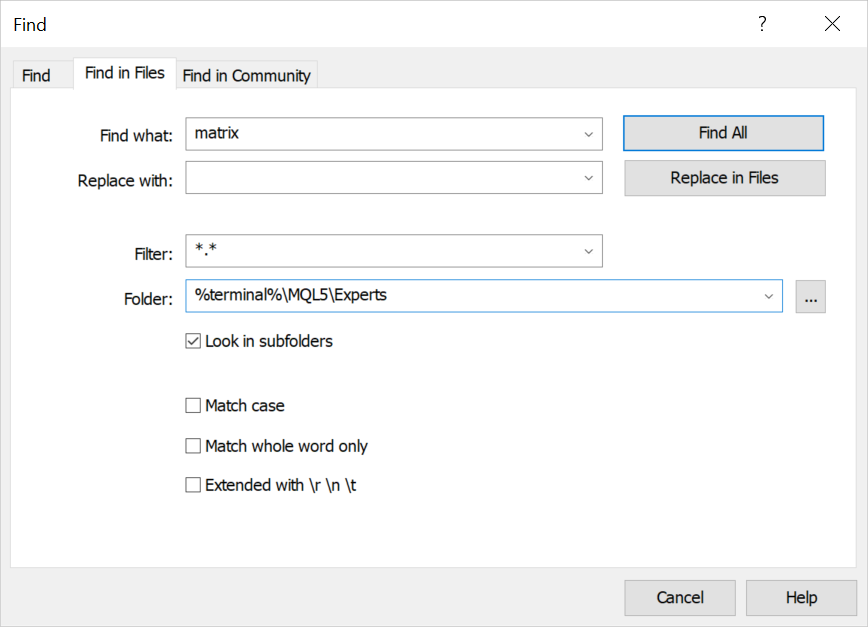22. MetaEditor: Improved display of arrays in the debugger.
23. MetaEditor: Increased buffer for copying values from the debugger.
24. MetaEditor: Improved error hints.
25. MetaEditor: Added indication of relative paths in the *.mproj project file. Previously, absolute paths were used, which resulted in compilation errors in case the project was moved.
26. MetaEditor: Added automatic embedding of BMP resources as globally available 32-bit bitmap arrays in projects. This eliminates the need to call ResourceReadImage inside the code to read the graphical resource.
```'levels.bmp' as 'uint levels'
```
27. MetaEditor: Improved reading of extended BMP file formats.
28. MetaEditor: Updated UI translations.
29. Fixed errors reported in crash logs.

The update will be available through the Live Update system.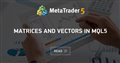Matrices and vectors in MQL5
• www.mql5.com
By using special data types 'matrix' and 'vector', it is possible to create code which is very close to mathematical notation. With these methods, you can avoid the need to create nested loops or to mind correct indexing of arrays in calculations. Therefore, the use of matrix and vector methods increases the reliability and speed in developing complex programs.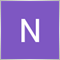14

The tester and meta editor features is still not working for Mac M1 and M2 if it is a fresh install. Old version works fine.

MT5 will freeze when trying to launch Meta Editor for few minutes then nothing happens. Running tester will also lead to a core stating "busy" then nothing happens.

This started in version 3300+ and is persisting.

There have been many similar complaints here: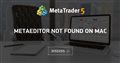• 2022.07.03
• www.mql5.com
Hello all, I've downloaded MT5 on my Mac, and for a few months I was able to run it properly and use MetaEditor...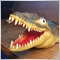698

I have an issue with StringFormat/PrintFormat with version 3360 as I described in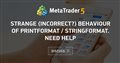Strange (incorrect?) behaviour of PrintFormat / StringFormat. Need help
• 2022.07.17
• www.mql5.com
Hi, today I stumbled across a very strange (or incorrect?) behaviour of the functions PrintFormat and/or StringFormat...698

In Build 3366:

Same issue as described in https://www.mql5.com/en/forum/428829Strange (incorrect?) behaviour of PrintFormat / StringFormat. Need help
• 2022.07.17
• www.mql5.com
Hi, today I stumbled across a very strange (or incorrect?) behaviour of the functions PrintFormat and/or StringFormat...698

Dr Matthias Hammelsbeck #:

In Build 3366:

Same issue as described in https://www.mql5.com/en/forum/428829

The same in Build 3367.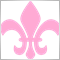57

When will mql5 introduce a Decimal type.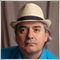11401

salitos #: When will mql5 introduce a Decimal type.

Probably never! After decades, they still have not learned their lesson about how to properly handle financial data.57

Fernando Carreiro #:

Probably never! After decades, they still have not learned their lesson about how to properly handle financial data.

lol. Cursory google search shows this https://github.com/vpiotr/decimal_for_cpp for example. It's bsd licensed so they could just add it to their C++ flavor if they do not want to do the heavy lifting.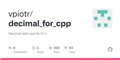GitHub - vpiotr/decimal_for_cpp: Decimal data type for C++
• vpiotr
• github.com
Decimal data type support, for COBOL-like fixed-point operations on currency/money values. Author: Piotr Likus Created: 03/01/2011 Modified: 19/07/2021 This data type is designed to perform calculation with on-fly roundings & to support correct compare function (floating-point compare is unreliable). Values are stored internally using 64-bit...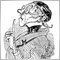Moderator
14155

Please correct the specifications of XAUUSD & XAGUSD (and provide hist. data for XAGUSD ).

Here are the results of calculating the optimal lot size if sl, risk an free margin are given: Gold and Silver with problems while EURUSD ok:

```MetaQuotes demo account (b. 3369 Beta channel)
2022.07.25 17:43:42.645 test_MM-functions (EURUSD,M1)   XAUUSD  in: 1717.65000 sl:1717.15000  diff:0.50000  delta:10.00000  delta:*diff:5.00000  tVal:0.10000  tSz:0.01000
2022.07.25 17:43:42.645 test_MM-functions (EURUSD,M1)   XAGUSD  in: 18.45100 sl:18.40100  diff:0.05000  delta:500.00000  delta:*diff:25.00000  tVal:0.50000  tSz:0.00100
2022.07.25 17:43:42.645 test_MM-functions (EURUSD,M1)   EURUSD  in: 1.02185 sl:1.02235  diff:0.00050  delta:97861.72139  delta:*diff:48.93086  tVal:0.97862  tSz:0.00001
2022.07.25 17:43:42.645 test_MM-functions (EURUSD,M1)   Set: Risk: 5.0 %, dSL: 50  Com/Lot: 2.00  Free: 9983.40 EUR:  XAUUSD  BU  in: 1717.65000  sl: 1717.15000  lotsC: 71.3100 (-3489.26)  lotsB: 10.2000 (-499.09)
2022.07.25 17:43:42.645 test_MM-functions (EURUSD,M1)   Set: Risk: 5.0 %, dSL: 50  Com/Lot: 2.00  Free: 9983.40 EUR:  XAGUSD  BU  in: 18.45100  sl: 18.40100  lotsC: 18.4900 (-4523.66)  lotsB: 2.0400 (-499.09)
2022.07.25 17:43:42.645 test_MM-functions (EURUSD,M1)   Set: Risk: 5.0 %, dSL: 50  Com/Lot: 2.00  Free: 9983.40 EUR:  EURUSD  SE  in: 1.02185  sl: 1.02235  lotsC: 9.8000 (-479.29)  lotsB: 10.2100 (-499.34)

RoboForex Demo account (b. 3320 release channel)
2022.07.25 17:46:22.772 test_MM-functions (EURUSD,D1)   XAUUSD  in: 1717.10000 sl:1717.15000  diff:0.05000  delta:100.00000  delta:*diff:5.00000  tVal:0.10000  tSz:0.00100
2022.07.25 17:46:22.772 test_MM-functions (EURUSD,D1)   XAGUSD  in: 18.43400 sl:18.43350  diff:0.00050  delta:5000.00000  delta:*diff:2.50000  tVal:0.05000  tSz:0.00001
2022.07.25 17:46:22.772 test_MM-functions (EURUSD,D1)   EURUSD  in: 1.02186 sl:1.02236  diff:0.00050  delta:100000.00000  delta:*diff:50.00000  tVal:1.00000  tSz:0.00001
2022.07.25 17:46:22.773 test_MM-functions (EURUSD,D1)   Set: Risk: 5.0 %, dSL: 50  Com/Lot: 2.00  Free: 5348.69 USD:  XAUUSD  SE  in: 1717.10000  sl: 1717.15000  lotsC: 38.2000 (-191.00)  lotsB: 53.4900 (-267.45)
2022.07.25 17:46:22.773 test_MM-functions (EURUSD,D1)   Set: Risk: 5.0 %, dSL: 50  Com/Lot: 2.00  Free: 5348.69 USD:  XAGUSD  BU  in: 18.43400  sl: 18.43350  lotsC: 59.4300 (-148.57)  lotsB: 106.9700 (-267.42)
2022.07.25 17:46:22.773 test_MM-functions (EURUSD,D1)   Set: Risk: 5.0 %, dSL: 50  Com/Lot: 2.00  Free: 5348.69 USD:  EURUSD  SE  in: 1.02186  sl: 1.02236  lotsC: 5.1400 (-257.00)  lotsB: 5.3500 (-267.50)```

Lot size of 71.31 and 18.49 are way to high. I attached the script to compare an alternative version of calculation. Thanks B. Flotterer: https://www.mql5.com/de/forum/394471/page1408#comment_41003325.

Here are the specs for Gold of RoboForex and MQ: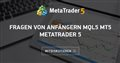Fragen von Anfängern MQL5 MT5 MetaTrader 5
• 2022.07.20
• www.mql5.com
Können Sie mir sagen, ob dies eine realistische Testerfigur ist...
Files:698

Dr Matthias Hammelsbeck #:

I have an issue with StringFormat/PrintFormat with version 3360 as I described in

In Build 3370 all works fine!

Thank you

Matthias27

There is a bug in the 3390 user interface: